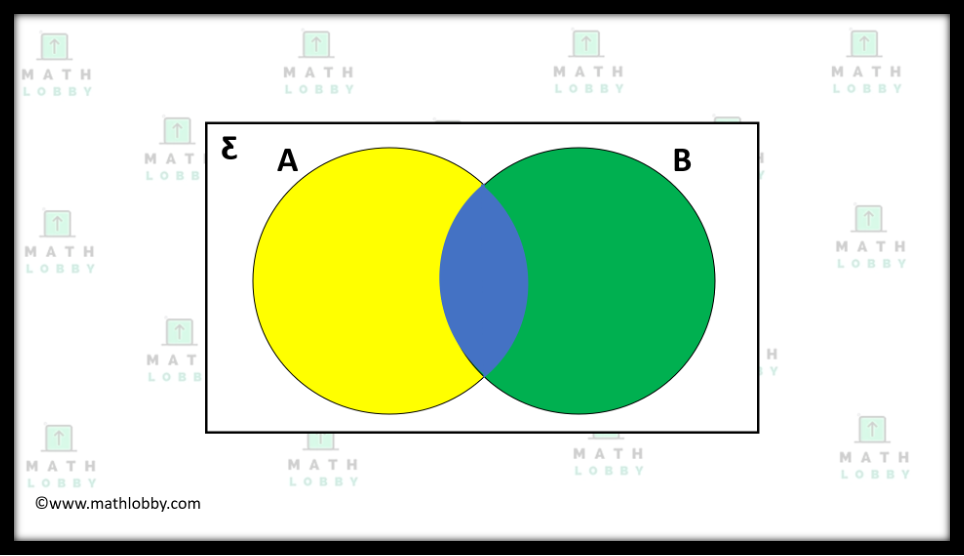top of page
Search

# Venn diagrams

Updated: Jun 22, 2021

Dear Secondary Math students, in our previous math article, we have covered set language and notations. It is necessary to understand the different set language and notations as we will be using them a lot in this chapter.

Please make sure that you understand and master it before proceeding to Venn Diagrams. Here is the link to our Set Language and Notations article.

By mathematical definition, Venn diagrams are “diagrams representing mathematical or logical sets pictorially as circles or closed curves within an enclosing rectangle (the universal set), common elements of the sets being represented by intersections of the circles.”

Let’s take a look at the diagram shown below:As you can see, sets A and B are denoted by two large circles in the diagram. The universal set, on the other hand, is denoted by the white rectangle containing sets A and B.

Set A is the large yellow circle while Set B is the green circle, which in between is the intersection between the sets, shown as the blue portion.

However, the diagram above is just for illustration purposes. In actual examinations, the circles will be white in color, and you will be asked to shade portions that represents the notation shown in the question.

*IMPORTANT: General rule of thumb when solving questions involving Venn Diagrams is to solve the question part by part.

What this means is to look at each of the sets from the question individually, then shade in a different direction for every part you solve, and depending on the notation, the resulting portion which crosses will be your answer! Remember to erase the portions which are not part of the answer!

Look at the example provided below:Another thing to note is that this does not work for every scenario, so do read the question carefully and approach the question flexibly!

Examples of some types of Venn diagrams and how they are shaded:The Venn diagram shown above represents the notation, “AB”, which is basically the set that includes all the elements from both A and B, hence both the circles must be shaded fully.The Venn diagram shown above represents the notation, “AB”, which is basically only the elements that exists in both set A and B, hence only the intersection between the circles must be shaded.The Venn diagram shown above represents the notation, “A’B”, which is basically only the intersection between the complement of A and set B, hence only strictly set B is being shaded, with the exclusion of the intersection between the two sets as well.The Venn diagram shown above represents the notation, “(A B)’”, which is basically the complement of the union of set A and set B, hence the whole rectangle is shaded except for the union of set A and set B.

And that’s all for today, students! Math Lobby hopes that after this article, you have a clear understanding and is equipped with the skills to deal with questions involving Venn diagrams!

If you have any pending questions, please do go on to our Facebook page, Instagram or contact us directly at Math Lobby! We have certified mathematics tutors to aid you in your journey to becoming a better student!

As always: Work hard, stay motivated and we wish all students a successful and enjoyable journey with Math Lobby!

If you want to receive more Secondary Math Tips from us,

Visit and FOLLOW our Instagram page at https://www.instagram.com/mathlobbymotivation/